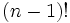# Quiz:Symmetric group

## Elements

 1,1,2,3,3 1,3,4,4,12 1,3,6,6,8 1,1,4,4,4,4,6 1,2,2,2,3,4,4,6

2 Given an element picked uniformly at random from symmetric group:S4, what is the probability that the square of this element is the identity element? See element structure of symmetric group:S4#Conjugacy class structure.

 1/4 5/12 1/2 7/12 2/3

3 What is the probability that two elements of symmetric group:S3, picked uniformly at random and independently (so they may be equal) generate the whole group? See generating sets for subgroups of symmetric group:S3.

 1/2 2/3 3/4 5/6 1

4 What is the smallest value of$n$ such that the symmetric group of degree$n$ contains an element of order 6?

 3 4 5 6 7

5 What is the maximum possible size of a conjugacy class in the symmetric group$S_n$ of degree$n$, for$n \ge 3$?$n!$$(n - 1)!$$(n - 2)!$$n!/(n - 1)$$n!/(n - 2)$